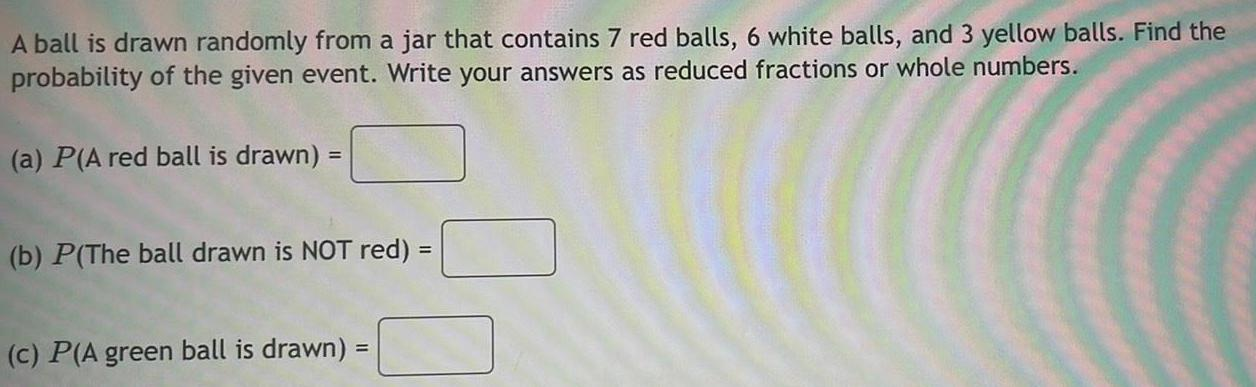Question:

# A ball is drawn randomly from a jar that contains 7 red

Last updated: 7/7/2022A ball is drawn randomly from a jar that contains 7 red balls, 6 white balls, and 3 yellow balls. Find the probability of the given event. Write your answers as reduced fractions or whole numbers. (a) P(A red ball is drawn) = (b) P(The ball drawn is NOT red) = (c) P(A green ball is drawn) =